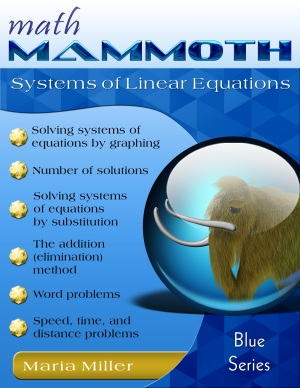Thanksgiving SALE! Get Math Mammoth and Make It Real Learning digital downloads at 30% off — right here at the MathMammoth.com website. Use coupon code THANKS30 at checkout. Offer valid through December 2, 2023.

^
You are here: HomeBlue Series → Systems of Linear Equations

# Math Mammoth Systems of Linear Equations103 pages

## Prices & ordering

Printed copy \$10.95

Math Mammoth Systems of Linear Equations is a worktext that covers how to analyze and solve pairs of simultaneous linear equations. This means students analyze and solve systems of linear equations that only contain two variables.

The first lesson, Equations with Two Variables, is optional. It reinforces the idea that a point on a line satisfies the equation of the line, and thus prepares the way for the main topics in the book.

First, students learn to solve systems of linear equations by graphing. Since each equation is an equation of a line, this is a simple technique, but it has its limitations, thus, algebraic solution methods will be taught also, in later lessons.

In the next lesson, we look at the number of solutions that a system of two linear equations can have. The three possible situations are easy to see based on the graphs of the equations: one solution (the lines intersect in one point), no solutions (lines are parallel), or an infinite number of solutions (the lines are the same).

In the next lesson, students learn the algebraic method of solving systems of equations by substitution. This is a straightforward technique; however, one has to be careful not to make simple mistakes. The lesson has some practice problems where students practice finding errors in solutions.

The next lesson, Applications, Part 1, has a variety of word problems that students can now solve using a system of equations. After that, students learn another algebraic method for solving systems of equations: the addition or elimination method. This is useful when the coefficients of the variables are such that you can easily find their least common multiple. Students also practice solving more complex systems, where the equations first have to be transformed and simplified, or include fractions.

Then it is time for more word problems, in the lesson Applications, Part 2. One lesson is devoted to problems about speed, time, and distance, and another for mixtures and comparisons. Making a chart is very helpful in these situations.

The book ends with a review lesson. The answers are appended.

The PDF version of this book can be filled in on a computer, phone, or tablet, using the annotation tools found in many PDF apps. See more.

## Better Yet - Bundle Deals!

*BONUS*: Buy any bundle listed above, and get the fully upgraded Soft-Pak software for FREE (includes 4 math and 2 language arts programs, complete with on screen and printable options).

## How and where to order

You can buy Math Mammoth books at:

• Here at MathMammoth.com website — simply use the "Add to cart" buttons you see on the product pages.
• Rainbow Resource carries printed copies for the Light Blue series books, plus several CDs (Light Blue and Blue series).
• Homeschool Buyers Club offers download versions of the Light Blue series, plus the Blue series bundle.
• Homeschool Planet sells the digital versions of the main curriculum and lesson plans to go with them.
• Lulu sells printed copies for most of the Math Mammoth materials (various series).

By purchasing any of the books, permission IS granted for the teacher (or parent) to reproduce this material to be used with his/her students in a teaching situation; not for commercial resale. However, you are not permitted to share the material with another teacher.

In other words, you are permitted to make copies for the students/children you are teaching, but not for other teachers' usage.

Math Mammoth books are PDF files. I recommend you use Adobe Reader to view them, including if you use a Mac. You can try other PDF viewers, but they may at times either omit or mess up some of the images.
WAIT!

Receive my monthly collection of math tips & resources directly in your inbox — and get a FREE Math Mammoth book!You can unsubscribe at any time.

### Math Mammoth Tour

Confused about the different options? Take a virtual email tour around Math Mammoth! You'll receive:

An initial email to download your GIFT of over 400 free worksheets and sample pages from my books. Six other "TOURSTOP" emails that explain the important things and commonly asked questions concerning Math Mammoth curriculum. (Find out the differences between all these different-colored series!)

This way, you'll have time to digest the information over one or two weeks, plus an opportunity to ask me personally about the curriculum.
A monthly collection of math teaching tips & Math Mammoth updates (unsubscribe any time)### "Mini" Math Teaching Course

This is a little "virtual" 2-week course, where you will receive emails on important topics on teaching math, including:

- How to help a student who is behind
- Troubles with word problems
- Teaching multiplication tables
- Why fractions are so difficult
- The value of mistakes
- Should you use timed tests
- And more!

A GIFT of over 400 free worksheets and sample pages from my books right in the very beginning.A monthly collection of math teaching tips & Math Mammoth updates (unsubscribe any time)
Enter your email to receive math teaching tips, resources, Math Mammoth news & sales, humor, and more! I tend to send out these tips about once monthly, near the beginning of the month, but occasionally you may hear from me twice per month (and sometimes less often).• A GIFT of over 400 free worksheets and sample pages from my books.